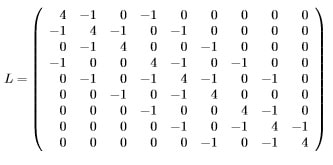# Mathematics 719, Applied Linear Algebra Assignment 1

Include all written answers, tables, graphs, images and MATLAB code for each problem. Send a separate .m file for each question where you are requested to do so. Also remember to send along any auxiliary .m files that are necessary to run your code. I should be able to run your code without any modifications so as to get the same results you include with this assignment. Make sure that the code you submit is your own.

1. In this problem we endeavour to establish the limits on the size (n × n) of systems of equations that we may reasonably solve. When the questions below ask about your computer they refer to one of the computers available to you in the computer labs at Ryerson. You may develop the programs necessary to test and prove your assertions on any computer you like, but the final results should be about one of the lab computers.

• In which lab was your computer located?
• What is the largest square matrix that you can create on your computer with Matlab?
• How long does it take for you to solve a (dense) 100×100, 1000×1000, 10,000×10,000, system of equations (in seconds)?
• If your computer had sufficient memory, predict how long it would take to solve a 100,000×100,000 system of equations.
• Given enough memory estimate what is the largest n × n system you could solve in 24 hours.
1. For a given positive integer n we write a Matlab function to calculate the n2 × n2 discrete Laplacian matrix (see page 565 of the text for details).
1. Write a Matlab function whose prototype is L = discreteLaplacian(n), where n determines the size (n2× n2) of the discrete Laplacian matrix L. For example, if n = 3 then